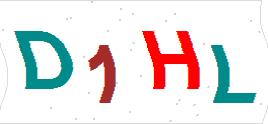不锈钢冲压对焊弯头，GB/T12459对焊管件，45度，90度，180度对焊式弯头不锈钢冲压对焊弯头，GB/T12459对焊管件，45度，90度，180度对焊式弯头，主要用于化工，石化，机电，船舶，机械制造，气动，液压管道等各种各样的场合，不锈钢冲压对焊弯头，GB/T12459对焊管件，45度，90度，180度对焊式弯头，材质有304，316等，不锈钢材料耐腐蚀，耐压高等

DN15=1/2A系列21.3B系列18SCH5S=1.6SCH10S=2.1SCH20S=2.6SCH40=2.9SCH80=3.6SCH160=4.5

DN20=3/4A系列26.9B系列25SCH5S=1.6SCH10S=2.1SCH20S=2.6SCH40=2.9SCH80=4.0SCH160=5.6

DN25A系列33.7B系列32SCH5S=1.6SCH10S=2.8SCH20S=3.2SCH40=3.2SCH80=4.5SCH160=6.3

DN32A系列42.4B系列38SCH5S=1.6SCH10S=2.8SCH20S=3.2SCH40=3.6SCH80=5.0SCH160=6.3

DN40A系列48.3B系列45SCH5S=1.6SCH10S=2.8SCH20S=3.2SCH40=3.6SCH80=5.0SCH160=7.1

DN50A系列60.3B系列57SCH5S=1.6SCH10S=2.8SCH20S=3.6SCH40=5.0SCH80=5.6SCH160=8.8

DN65A系列76.1B系列76SCH5S=2.0SCH10S=3.0SCH20S=3.6SCH40=5.0SCH80=7.1SCH160=10

DN80A系列88.9B系列89SCH5S=2.0SCH10S=3.0SCH20S=4.0SCH40=5.6SCH80=8.0SCH160=11

DN100A系列114.3B系列108SCH5S=2.0SCH10S=3.0SCH20S=4.0SCH40=5.9SCH80=8.8SCH160=14.2

DN125A系列139.7B系列133SCH5S=2.9SCH10S=3.4SCH20S=5.0SCH40=7.0SCH80=11SCH160=16

DN150A系列168.3B系列159SCH5S=2.9SCH10S=3.4SCH20S=5.6SCH40=7.0SCH80=12.5SCH160=17.5

DN200A系列219.1B系列219SCH5S=2.9SCH10S=4.0SCH20S=6.3SCH40=8.0SCH80=14.2SCH160=22.2

DN250A系列273B系列273SCH5S=3.6SCH10S=4.0SCH20S=6.3SCH40=8.8SCH80=17.5SCH160=28

DN300A系列323.9B系列325 SCH5S=4.0SCH10S=4.5SCH20S=6.3SCH40=10.0SCH80=17.5SCH160=32

DN350A系列355.6B系列377SCH5S=4.0SCH10S=5.0SCH20S=8.0SCH40=11SCH80=20SCH160=36

1定货时要说明管件的形式，种类，规格，材质，型号，压力等级，管子是A系列，还是B系列，管子的壁厚，是加长的，还是短的，等等具体参数，以便选型和报价

2冲压对焊管件运输时不要损坏焊接坡口，会导致焊接时不均

3不锈钢冲压对焊管件外表面一般是酸洗，外观美观，运输安装时不要损坏内外表面

4不锈钢对焊管件耐腐蚀，耐压高，是一种较为理想的管道配件点击换一张

2.如有必要,请您留下您的详细联系方式!

 不锈钢冲压对焊弯头，GB/T12459对焊管件，45度，90度，180度对焊式弯头 GB/T12459 精品打造304，316不锈钢,DN32,PN6,HG20593化工部标准平焊板式法兰，HG20594带颈平焊法兰，HG20595带颈对焊法兰 HG20593,HG20594,HG20595 精品打造304，316不锈钢DN100,PN16,GB/T9119国家标准平焊板式法兰，GB/T9116带颈平焊法兰，GB/T9115带颈对焊法兰 GB/T9119,GB/T9116,GB/T9115 精品打造304，316不锈钢GB/T12459,B16.11,DN100冲压对焊接式45°，90°，180°度长半径1.5D弯头管件 GB/T12459,S90EL 精品打造304，316不锈钢GB/T12459,B16.11冲压对焊接式等径异径三通，TS,TR对焊式三通管件 GB/T12459,TS,TR